This article is my interpretation of Tanuj Khatta’s awesome tutorial. I’ll change some things in my explanation, but he did an incredible job building the structure of his text and I’ll try to follow it.

The centroid decomposition is a very simple idea that is able to solve some really scary problems. In fact, you only need to know depth first search to understand it.

I’ll split this article into three pieces. First, we’ll see an example of a problem that the centroid decomposition tries to solve. Then, we’ll define what a centroid and a centroid decomposition are. The last, and most challenging part, will be exploring the properties of this technique.

If you have any suggestions or questions, please don’t be shy. Comment here or tell me on Twitter.

Problem description

The problem Xenia and Tree will guide our discussion about centroid decomposition.

In this problem, we have a tree with colored nodes. Initially, the first node is painted in rose, and the others ones, in blue.

After reading the tree, we have to process two types of operations:

• update(a): Change the color of the blue node a to rose.
• query(a): Find the distance from a to the closest rose node.

A naive solution

The first idea is, for each query, do a BFS/DFS to find the closest rose node. Then, for each update, you just change the value of the array storing the color of the node.

This strategy allows an update in O(1) time complexity, at the cost of having a query in O(n).

Another naive solution

It’s also possible to invert the logic behind the last solution.

Now, we maintain an array ans such that ans[a] is the distance from a to the closest rose node. We can initialize that array with the distance from all nodes to the first one, because it’s always rose at the beginning.

For each query, we just return the value of ans[a] in O(1) time complexity, and, for each update, we just run a BFS/DFS over the tree to change the value of ans when necessary in O(n).

The first solution has a good update, but a terrible query. The second one has a good query, but a terrible update. The natural thing to do now is ask yourself if is there a way to have both operations balanced.

What is a centroid?

Given a tree with n nodes. Let’s define the centroid of that tree as a node a such that all trees resulting from the removal of a has at most n/2 nodes.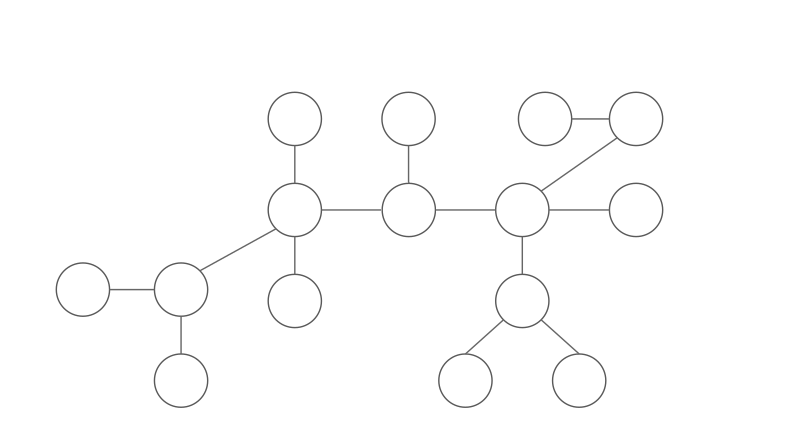Animation #1: Example of a centroid node.

Please, don’t confuse the centroid with the center of a tree. We can define the center of a tree in three equivalent ways:

1. Intuitive way: The node(s) such that, if root(s), minimize the height of the rooted tree.

2. Algorithmic way: The node(s) in the middle of the largest path in the tree.

3. Formal way: The set of nodes that minimize the maximum distance between them and any other node in the tree.

The concept of center and centroid are very similar, but they were made to represent different ideas. The center is related to the height of the tree, while the centroid would be equivalent to its center of mass.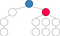Figure #1: The blue node is the center of the tree, while the rose node is its centroid.

Is there always a centroid?

Yes. Every tree has at least one centroid.

How can we find the centroid of a tree?

Initially, choose any vertex a.

If a is a centroid, nice, we’re done. If not, there is one, and exactly one, component (a tree resulting from the removal of a) with more than n/2 nodes. Let b be the neighbor of a in that component.

Apply, now, the same argument recursively for node b.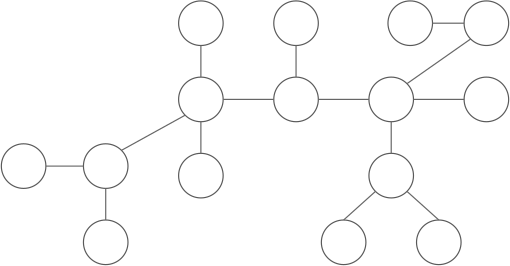Animation #2: Execution of the algorithm to find the centroid of a tree.

This algorithm works because it doesn’t visit a visited node or, in other words, it doesn’t go back from b to a. Suppose, by contradiction, it does. It’d visit a component with less than n/2 nodes, absurd.

The time complexity of this algorithm is O(n). We first run a DFS to evaluate the size of the subtree rooted at each node and, then, run another DFS to actually find the centroid.

Exercises

1. Show that a tree has at most one centroid, or give a counterexample.

2. In what class of trees are the center and centroid the same?

What is a centroid decomposition?

The centroid decomposition of a tree is another tree defined recursively as:

• Its root is the centroid of the original tree.
• Its children are the centroid of each tree resulting from the removal of the root from the original tree.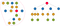Figure #2: The centroid decomposition (on the right) of a tree.

(You may want to think about exercises 3 and 4 right now. It’ll surely develop your intuition about centroid decomposition.)

Implementation

It’s not difficult to understand the implementation of centroid decomposition. The idea is simply to apply the definition using a DFS.

Time complexity

It’s not hard to see that the complexity of building the centroid decomposition is O(n²). In the end, each one of the n nodes will become the centroid of a tree and we’ll need to run one DFS on each one of those trees to find each one of those n centroids.

What is a little bit more difficult to understand is that the complexity is, indeed, O(n lg(n)). Each node is the centroid of a tree, of course, but the size of that tree is getting smaller and smaller.

As a matter of fact, its analysis is pretty similar to the merge sort one. If we look at the time to build each level of the centroid decomposition, we’ll see that the first level is made in O(n) time complexity, because we just find the centroid of the whole tree.

Let’s look at the second level of the centroid decomposition. It’s hard to say exactly what is the time complexity to find each one of the centroids in that level, but we know that the sum of the tree’s size in that level is n-1 (why?). It means that the complexity to build all the centroids of the second level is O(n). The same argument goes for the other levels.

We can conclude that the time complexity to build the centroid decomposition is O(hn) where h is the height of the centroid decomposition. It’s easy to see that h = lg(n) (look at the size of the tree in each level).

You may be asking yourself if removing the edges of the tree, like we did in the implementation, may affect the complexity somehow. It doesn’t. There are n-1 edges to be removed, each one in O(lg(n)). The total time we spend doing that is also O(n lg(n)).

Properties of the centroid decomposition

1. A vertex belongs to the component of all its ancestors.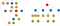Figure #3: The node 14 belongs to the component of 14, 15, 11 and 3.

Proof: It’s easier if you think about the descendants instead of ancestors. The node a is a descendants of a node b if a belongs to the tree resulting from the removal of b. Note that the removal of b will only affect its own component, because we erased the edge from b to its dad in the centroid decomposition (see the implementation for the details). It means that all the descendants of b belongs to its component. ∎

2. The path from a to b can be decomposed into the path from a to lca(a,b) and the path from lca(a,b) to b, where lca(a,b) is the lowest common ancestor of a and b in the centroid decomposition.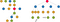Figure #4: The path from 9 to 10 in the original tree can be decomposed into the path from 9 to 3 and the path from 3 to 10.

Proof: Both a and b belong to the component where the node lca(a,b) is the centroid. Suppose, by contradiction, that lca(a,b) doesn’t divide the path from a to b into two disjoint parts.

It means that both a and b will be in the same component after the removal of lca(a,b) in the original tree. Consequently, the centroid of that component would be a common ancestor of a and b lower than lca(a,b). Absurd. ∎

3. Each one of the n² paths of the original tree is the concatenation of two paths in a set of O(n lg(n)) paths from a node to all its ancestors in the centroid decomposition.

That’s the most important and the hardest idea to understand! Let’s see an example first. Look at the node 14 on figure 3. We have n paths starting in node 14 and ending in node a. We can represent all those paths in four different ways:

1. a ϵ {14} ➡ from 14 to 14 and then from 14 to a.

2. a ϵ {15} ➡ from 14 to 15, and then from 15 to a.

3. a ϵ {6, 9, 13} ➡ from 14 to 11, and then from 11 to a.

4. a ϵ {1, 2, 4, 5, 7, 8, 10, 12} ➡ from 14 to 3, and then from 3 to a.

Note that 14, 15, 11 and 3 are the ancestors of 14 in the centroid decomposition. What is the relation between a and them?

The idea is that instead of choosing all possible endpoints of the path in the original tree, we’ll choose all the lowest common ancestors of two nodes in the centroid decomposition.

This technique allows us to represent n paths using just two paths in a set of four ways. If we generalize the idea to any node, we can represent n² paths using two paths in a set of O(n lg(n)) ways.

Proof: The last property says that each path in the original tree can be represented as two in the centroid decomposition (from a to lca(a,b) and from lca(a,b) to b). Now, we have to prove that there’s only O(n lg(n)) paths from every node to its ancestors.

The height of the centroid decomposition is logarithmic. It means that the number of ancestors of a node is O(lg(n)). There are n nodes, so the total number of ancestors is O(n lg(n))∎

Another proof: The last property says that each path in the original tree can be represented as two in the centroid decomposition (from a to lca(a,b) and from lca(a,b) to b). Now, we have to prove that there’s only O(n lg(n)) paths from every node to its ancestors.

Instead of looking at all the ancestors of a node, let’s look at all its descendants (the number of ancestors has to be equal to the number of descendants). We’ll analyze, again, each level of the centroid decomposition.

It’s easy to see that the root has n-1 descendants.

Again, we don’t know how many descendants each node of the second level has. We know, nonetheless, that the sum of descendants of all nodes in the second level is O(n). The same goes for other levels.

For each one of the log(n) levels, we have no more than n descendants. It means that the number of descendants / ancestors is O(n lg(n)). ∎

Exercises

3. Given the centroid decomposition of figure 5, find the original tree. Is there more than one possible answer?

4. Show that every centroid decomposition is a centroid decomposition of itself or give a counterexample.

5. Think about the following statement: “Given any rooted tree, the path from a to b can be decomposed into the path from a to lca(a,b) and the path from lca(a,b) to b and we can apply the same strategy used in the third property.” If that’s true, why should we use centroid decomposition?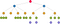Figure #5: Centroid decomposition for exercise 1.

Problems analysis

We can now solve the problem Xenia and Tree.

Let ans[a] be the distance to the closest rose node to a in the component where node a is centroid. Initially, ans[a] = ∞ because all nodes are blue (we’ll update the first node before reading the operations).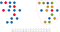Figure #6: Example of a painted tree (on the left), the components of each centroid (on the right) and the ans array.

Update

For each update(a), we do ans[b] = min(ans[b], dist(a, b)) for every ancestor b of a, where dist(a,b) is the distance in the original tree.

We know that a node is present in the component of all its ancestors, so we are just maintaining the property of ans array.

The time complexity of update is O(lg²(n)) because we are moving up on the tree of height lg(n) and for each step we evaluate dist(a,b) in O(lg(n)).

Query

For each query(a), we take the minimum of dist(a,b)+ans[b] for every ancestor b of a, where dist(a,b) is the distance in the original tree.

Let c be node corresponding to ans[b] for each ancestor b of a. We are decomposing the path from a to c into two parts:

1. From a to b: dist(a,b)
2. From b to c: ans[b]

Such that dist(a,b)+ans[b] is the distance from a to the closest rose node in the component where b is centroid.

We are looking at the answer of a subtree that is getting bigger and bigger at each iteration, until the subtree is the tree itself (when we look at the root of the centroid decomposition).

The time complexity of the query is exactly the same of the update, for the same reasons.

Written by

Igor Carpanese

A publication about Computer Science and Mathematics.

Welcome to a place where words matter. On Medium, smart voices and original ideas take center stage - with no ads in sight. Watch
Follow all the topics you care about, and we’ll deliver the best stories for you to your homepage and inbox. Explore
Get unlimited access to the best stories on Medium — and support writers while you’re at it. Just \$5/month. Upgrade